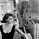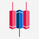# Don't miss the great buy opportunity in NGAS

Long
CURRENCYCOM:NATURALGAS   Natural Gas
319 views
Midterm forecast:
While the price is above the support 1.400, beginning of uptrend is expected.
We make sure when the resistance at 2.250 breaks.
If the support at 1.400 is broken, the short-term forecast -beginning of uptrend- will be invalid.

Technical analysis:
The RSI bounced from the support #1 at 30 and it prevented price from more losses.
While the RSI downtrend #2 is not broken, bearish wave in price would continue.
A trough is formed in daily chart at 1.640 on 07/20/2020, so more gains to resistance(s) 1.900, 2.000, 2.100 and maximum to Major Resistance (2.250) is expected.
Price is above WEMA21, if price drops more, this line can act as dynamic support against more losses.
Relative strength index ( RSI ) is 58.

. There is a possibility of temporary retracement to suggested support zone (1.650 - 1.550). if so, traders can set orders based on Price Action and expect to reach short-term targets.

Take Profits:
TP1= 1.900
TP2= 2.000
TP3= 2.100
TP4= 2.250
TP5= 2.500
TP6= 2.700
TP7= 2.950
TP8= 3.650
TP9= Free
Total Profit: 473

Update Details:

Closed Profit:
TP2 @ 2.00 touched at 2020.08.03 with 6 point Profit.
TP3 @ 2.10 touched at 2020.08.03 with 16 point Profit.
TP4 @ 2.25 touched at 2020.08.04 with 31 point Profit.
TP5 @ 2.50 touched at 2020.08.17 with 56 point Profit.
TP6 @ 2.70 touched at 2020.08.27 with 76 point Profit.
6 + 16 + 31 + 56 + 76 = 185

Open Profit:
Profit for one trade is 2.66(current price) - 1.94 (open price) = 72 point
4 trade(s) still open, therefore total profit for open trade(s) is 72 x 4 = 288 point
All SLs moved to Break-even point.

Take Profit:
TP7= 2.950
TP8= 3.350
TP9= 3.700
TP10= Free
Total Profit: 629

Update Details:

Closed Profit:
TP2 @ 2.00 touched at 2020.08.03 with 6 point Profit.
TP3 @ 2.10 touched at 2020.08.03 with 16 point Profit.
TP4 @ 2.25 touched at 2020.08.04 with 31 point Profit.
TP5 @ 2.50 touched at 2020.08.17 with 56 point Profit.
TP6 @ 2.70 touched at 2020.08.27 with 76 point Profit.
TP7 @ 2.95 touched at 2020.09.24 with 111 point Profit.
6 + 16 + 31 + 56 + 76 + 111 = 296

Open Profit:
Profit for one trade is 3.05(current price) - 1.94 (open price) = 111 point
3 trade(s) still open, therefore total profit for open trade(s) is 111 x 3 = 333
All SLs moved to Break-even point.

Take Profit:
TP8= 3.350
TP9= 3.700
TP10= Free
HighAccurate forecast for
★36 Forex Pairs: 916.000 pips track record profit
★34 Cryptos
★Gold, Silver, Oils, Gas

in 9 languages

since 2007

http://www.ForecastCity.com
★FREE Telegram http://t.me/forecastcity_com